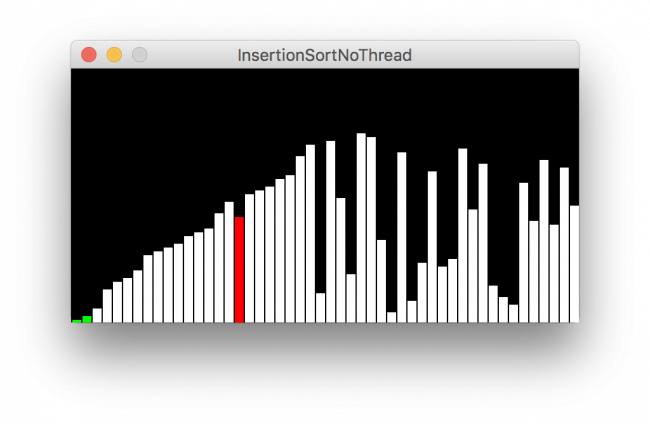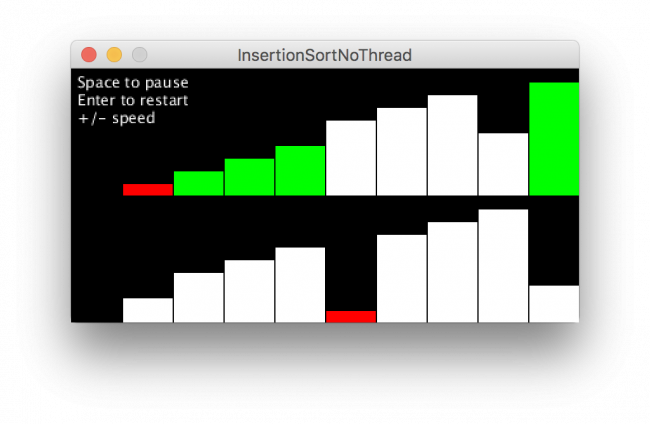#### Howdy, Stranger!

We are about to switch to a new forum software. Until then we have removed the registration on this forum.

# Updating outside of draw()

edited March 2017

I am trying to make visualizations of common sorting algorithms using processing. I would like to be able to call the draw function after every step the algorithm takes, so that you can see everything as it happens. Here is an example of what i am trying to do with Insertion sort:

`````` public void insertionSort()
{
for(int i = 0; i < arr.length; i++)
{
while(j > 0 && arr[j-1] > arr[j])
{
swap(arr, j, j-1);
j--;
draw();
}
j = i;
}
}

public void draw()
{
(draw everything)
}
``````

I know for simpler sorts like selection and insertion sort you could make the sort work within the draw function itself, which i have done, but that wont work for sorts that use recursion like quick sort and merge sort (at least i cant think of how to get them to work). So basically, my question is is there any way to choose when the draw function executes so i can call it and have it update when i want? I have tried the redraw() function but that doesn't seem to be fast enough, and simply calling draw doesn't do anything either. I also tried running the sort in a separate thread, which sort of works but has some bad side effects with the screen glitching out.

Tagged:

• You have to learn how draw works to use it to your advantage. Here is an example below.

https://processing.org/reference/draw_.html

Kf

``````String[] src = { "deer", "elephant", "bear", "aardvark", "cat", "eagle", "TRex", "Cougaar" };

String[] dest;
int n=1;

void setup() {
size(800, 200);
textAlign(CENTER, CENTER);
fill(0);
frameRate(1);

}

void draw() {
background(255);

dest = new String[n];
arrayCopy(src, dest, n);
dest = sort(dest);

for (int i=0; i<n; i++)
text(""+dest[i],(i+1)*width/float(n+1), height/2);
n++;

if(n==src.length+1){
text("DONE",width/2,height/4*3);
noLoop();

}
}
``````
• I would like to be able to call the draw function after every step the algorithm takes

draw() is also the animation clock for a Processing sketch (frameRate etc.) so what you probably want instead is an object that encapsulates a sort algorithm and its state, eg `BubbleSort bsort`

Declare the object, then in draw call `bsort.next()` to advance the state, then draw the updated state to the screen using `bsort.arr`etc.

• Thanks for the responses guys. I actually figured out that i can in fact run the algorithm perfectly fine in separate thread as long as i delay long enough in between each step for the draw function clear and redraw everything. Don't know why i didn't try that the first time. Thanks for the help though.

• Interesting! Willing to share a code example for that approach?

• Sure! Here is the full sketch for the insertion sort animation:

``````import java.util.Random;
int N = 50;
int[] arr;
int i;
int j;
int w;
int h;

void setup()
{
size(1000,800);
//initialize variables
i = 0;
w = width/N;
h = (height-50)/N;

//make the array and ititialize it with values
arr = new int[N];
for(int i = 0; i < N; i++)
arr[i] = i;

//make an instance of random
Random random = new Random();
random.nextInt();

//shuffle the array
for (int i = 0; i < N; i++)
{
int change = random.nextInt(N);
swap(arr, i, change);
}

//run the insertion sort in a separate thread
}
//insertion sort
void insertionSort()
{
for(int i = 0; i < arr.length; i++)
{
for(int j = i; j > 0; j--)
{
this.j = j - 1;
if(arr[j-1] > arr[j])
swap(arr, j, j-1);
else
break;

//delay after each step so draw has time to update the screen
delay(50);
}
}
}

void draw() {
background(0);

//show the blocks
for(int i = 0; i < arr.length; i++)
{
//if its the counter make it red
if(i == j)
{
fill(255,0,0);
rect(i * w, height, w, -(arr[i] * h + h));
}
//if its in the correct spot make it green
else if(arr[i] == i)
{
fill(0,255,0);
rect(i * w, height, w, -(arr[i] * h + h));
}
//else make it white
else
{
fill(255);
rect(i * w, height, w, -(arr[i] * h + h));
}
}
}

void swap(int[] a, int i, int change) {
int helper = a[i];
a[i] = a[change];
a[change] = helper;
}
``````
• @isaac328 -- thank you for sharing this use of `thread()` combined with reading global variables to render the current state of nested loops.

I like that your approach can monitor sorting code as-is, from standard examples!

Here is a quick example of an alternate object-based approach which does not use `thread()` or `Thread`. This makes it easier to incorporate interactive control over changing the running speed, pausing, manually advancing, switching between algorithms etc. etc.

A Sorter is a class. An InsertionSorter defines the step method for that class. Each call to `iSort.step()` advances the array to the next Insertion Sort state. You can create multiple sorters and multiple sort displays.

``````/** InsertionSort with no thread
* 2017-04-02 - Jeremy Douglass - Processing 3.2.3 - based on a sketch by isaac328
* forum.processing.org/two/discussion/21696/updating-outside-of-draw
**/
int N = 10;
boolean pause;
InsertionSorter iSort;
InsertionSorter iSort2;

void setup() {
size(400, 200);
iSort = new InsertionSorter(N, width, height/2);
iSort2 = new InsertionSorter(N, width, height/2);
frameRate(21);
}

void draw() {
if (!pause) {
background(0);
iSort.step();
iSort.render(0,0);
if(iSort2.sorted){ iSort2.reset(); }
iSort2.step();
iSort2.render(0,height/2);
text("Space to pause\nEnter to restart\n+/- speed", 5, 15);
}
}

void keyReleased() {
// pause
if (key==' ') {
pause = !pause;
}
// restart
if (!pause && keyCode==ENTER) {
iSort.reset();
iSort2.reset();
}
if (!pause && key=='-' && frameRate > 10) {
frameRate(frameRate-10);
}
if (!pause && key=='=' || key=='+') {
frameRate(frameRate+10);
}
}

class Sorter {
int[] arr;
int i, j, swap;
int w, h, size;
boolean sorted;
Sorter(int size_, int w_, int h_) {
size = size_;
arr = new int[size];
w = w_/size;
h = h_/size;
// values
for (int i = 0; i < size; i++) {
arr[i] = i;
}
shuffle();
}
void render(float x, float y) {
pushMatrix();
translate(x,y);
for (int i = 0; i < arr.length; i++) {
pushStyle();
if (i == j) {           // if it is the counter
fill(255, 0, 0);      // make it red
}
else if (arr[i] == i) { // if in the correct spot
fill(0, 255, 0);      // make it green
}
else {
fill(255);            // else make it white
}
rect(i * w, (h * size), w, -(arr[i] * h));
popStyle();
}
popMatrix();
}
void reset() {
i = j = swap = 0;
shuffle();
sorted = false;
}
void shuffle(){
IntList list = new IntList(arr);
list.shuffle();
arr = list.array();
}
}

class InsertionSorter extends Sorter {
InsertionSorter(int size, int w_, int h_) {
super(size, w_, h_);
}
void step() {
if (j>0) { // item unsorted
if (arr[j-1] > arr[j]) { // item swap
swap = arr[j];
arr[j] = arr[j-1];
arr[j-1] = swap;
j--; // next item comparison
} else { // item done
j=-1;
return;
}
}
if (j<1) { // need new item
if (i<arr.length-1) { // next item
i++;
j=i;
} else { // array all sorted
sorted = true;
return;
}
}
}
}
``````• Thinking about these approaches a bit more, I think that having multiple algorithms that each perform exactly the same number of steps (rather than being in racing threads) would be particularly useful if trying to do side-by-side comparison animations, as for example on this page:

• No offence, but delaying 50 milliseconds for every step of the algorithm is kind of pointless to put it in a seperate thread.

• @Lord_of_the_Galaxy -- looking back at this, I think there is one way that the thread approach has a real advantage for this kind of code. If you are using a lot of standard sorting algorithms, you can just "cut-paste" those algorithms into a threaded function that saves its state in global variables, add a delay, and animate from draw() -- no rewriting necessary. That is pretty convenient.

The class-based solution I shared as an alternative has many advantages -- starting / pausing / stopping, speed control, multiple objects, etc.... but it requires you to partly rewrite most algorithms to unwind them into a steps-with-states rather than nested loops.

• Perhaps you may be right. But I guess that's just your personal preference.